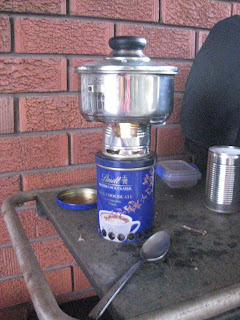## Saturday, 4 August 2012

### Stove output test

To get some idea of how much heat my EverythingNice stove outputs, I did a simple test. I placed a pot of water on top of it and measured the temperature of the water each minute.

The mass of the steel pot is 308g (without the lid), and contains 650g of water. The stove was lit, and after the flame settled down the pot was placed on top.The setup

Each minute the temperature of the pot was measured with an infrared thermometer. It was measured near the corner of the pot from the inside.Temperature vs Time

The graph from 2 -> 20min is fairly linear, so let's use that part of the graph. At 2min it was 24.8°C, at 20min it was 73.8°C.

ΔTemp = 73.8°C - 24.8°C = 49.0°C

The specific heat capacity of water is 4.18J/g.K, so the amount of energy that's gone into the water is

ΔEwater = 4.18J/g.K * 650g * 49K = 133.1KJ

And the specific heat capacity of steel is 0.47J/g.K, so the amount of energy that's gone into the steel is

ΔEpot = 0.47/g.K * 308g * 49K = 7.1KJ

So the total energy into our system is 133.1KJ + 7.1KJ =  140.2KJ

Now as

Δtime = 20min - 2min = 18min = 1080seconds

our power is 140.2KJ / 1080s = 130W

So it seems we put around 130W into our system, meaning our stove outputs at least that much. How much heat got "lost" ... I've no idea, but it gives us an idea of the sort of numbers we're looking at.